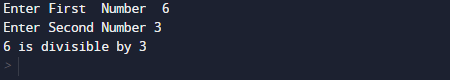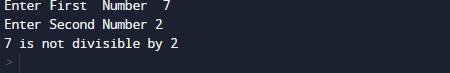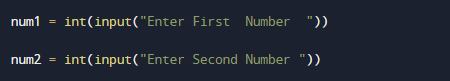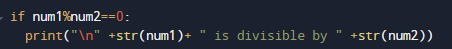# Python Program to Find Numbers Divisible by Another Number

In this tutorial you will learn about the Python Program to Find Numbers Divisible by Another Number and its application with practical example.

In this tutorial, we will learn to create a Python Program to Find Numbers Divisible by Another Number using python Programming.

## Prerequisites

Before starting with this tutorial we assume that you are best aware of the following Python programming topics:

• Python Operator.
• Basic Input and Output
• Basic Python  programming.
• Python Data type.
• List in Python.
• Python in-built Function.

## Divisibility of a number ?

Divisibility of a number is said to be divisible by another number if the remainder of a given number is 0  ( num1 % num2 == 0 ).

Example: suppose a  number 10 and another number is 5 so (10 % 5 == 0) So 10 is Divisible by 5.

## Python Program to Find Numbers Divisible by Another Number

In this program we will Find  A number is Divisible by Another number or not . We would first declared and initialized the required variables. Next, we would prompt user to input the values  later we will check these two number is divisible or not.

## Output

DivisibleNot DivisibleIn the above program, we have first declared and initialized a set variables required in the program.

• num1 = it will hold Numerator value
• num2= it will hold Denominator Value

And in the next statement user will be prompted to enter values of Numerator (First number) and Denominator(Second number) and assigned to variable ‘num1‘ and ‘num2’ respectively.After that  user inputs let say 10 as first number, then press ‘enter’ key,and then enter second number say 5 now  we will  check whether the number are divisible by 10 or not,As shown in the image given below:If the above condition  satisfied means the number is Numerator is divisible by Denominator.If not that means Numerator is not divisible by Denominator.In our program we will test whether a number(entered by user) is divisible by another number or not.

In this tutorial we have learn about the Python Program to Find Numbers Divisible by Another Number and its application with practical example. I hope you will like this tutorial.